# Fractions On A Number Line | Good To Know | WSKG

##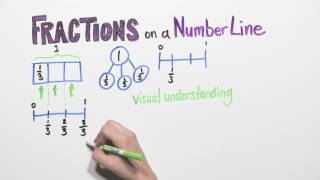By WSKG Public Media

Understand a fraction as a number on the number line; represent fractions on a number line diagram.# Comparing positive and negative numbers on the number line

##By Khan Academy

Comparing positive and negative numbers on the number line# Fractions and the Number Line - 3rd Grade

##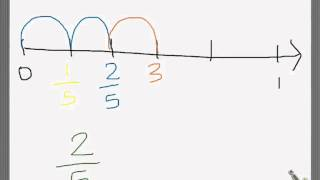By Hellothere1130

represent fractions on the number line# Plot Fractions on the Number Line

##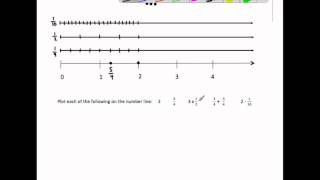By MathwithMrAlmeida

This video plots 6 fractions and operations with fractions on the number line. It ends by plotting all the same fractions on the number line and identify a pair of equivalent fractions and comparing each number's distance from zero.# Grade 3 Math #8.5, Fractions on a Number Line

##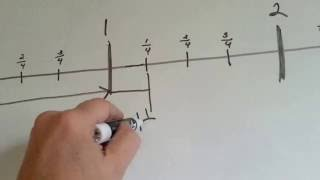By Joann`s School

How fractions are found on a number line. Whole numbers, mixed numbers, fractions on a number line# Negative numbers on the number line without reference to zero

##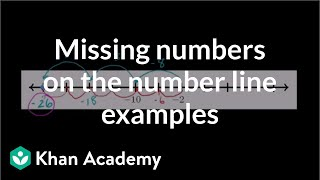By Khan Academy

Given a few negative numbers on a number line, let's see if we can determine what negative numbers are elsewhere on the number line.# Addition and subtraction with number lines

##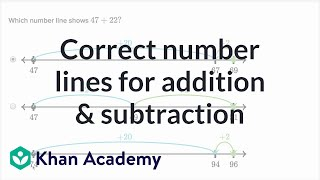By Khan Academy

Sal figures out which number line matches up with a 2-digit addition or subtraction problem.# Number line 1 | Multiplication and division | Arithmetic

##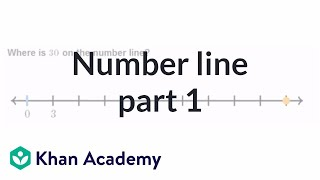By Khan Academy

Whole numbers on the number line# Fractions on a number line | Fractions | 3rd grade | Khan Academy

##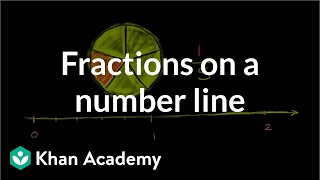By Khan Academy

Graph and locate fractions on a number line.##By Escholarindia Faridabad# Decimals on the number line 2

##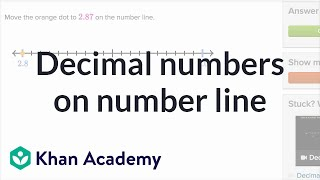By Khan Academy

Learn how to find decimals with hundredths on a number line.# Adding and subtracting on the number line word problems

##By Khan Academy

Adding and subtracting on the number line word problems# Fractions on a Number Line - Mr. Pearson Teaches 3rd Grade

##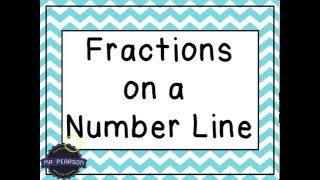By Mr Pearson teaches 3rd grade

This video is designed to teach students how to identify fractions on a number line.# Comparing fractions on a number line | Fractions | 4th grade | Khan Academy

##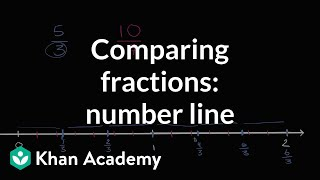By Khan Academy

Comparing fractions on a number line##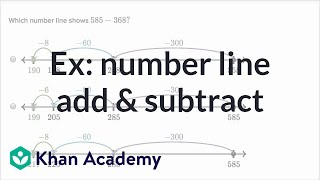By Khan Academy

Sal adds and subtracts numbers like 585 and 368 using a number line. Numbers used in these problems are less than 1000.# Inequalities on a number line

##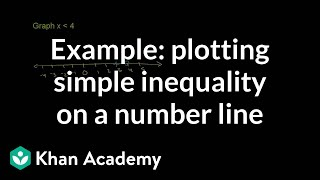By Khan Academy

Learn how to plot a simple inequality on a number line. The example used in this video is x < 4.# Plotting Fractions on a Number Line

##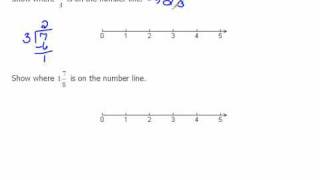By mathlablady4

The instructor first reviews how to plot whole numbers on a number line. She then relates the same concept to fractions. In the video lesson she plots both proper and improper fractions. Very clear instruction and easy for students to visualize the concept.# Algebra Lesson 4: Intervals and the Real Number Line

##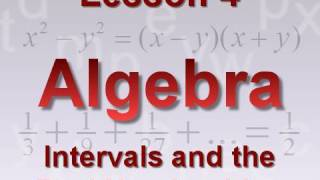By xoaxdotnet

YouTube presents Algebra Lesson 4: Intervals and the Real Number Line, an educational video resource on math.# Equivalent fractions using the number line (3.NF.3A)

##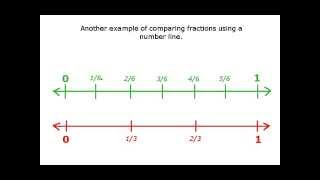By Far East Software

Understand two fractions as equivalent (equal) if they are the same size, or the same point on a number line.# Fractions on the Number Line

##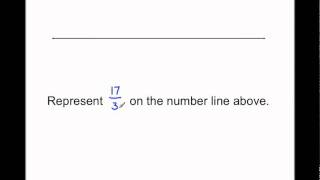By MathwithMrAlmeida

In this Singapore math lesson Mr. Almeida represents fractions on the number line by first defining how many parts make 1 whole. Then naming each part a fractional unit and counting that many units. This illustrates Common Core math standard 3.NF2.b.